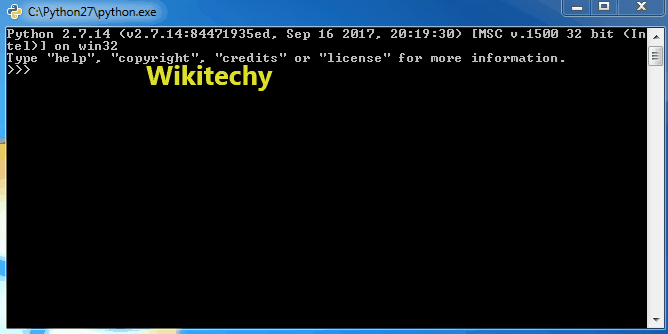# python tutorial - Python Global Variables | Global and Local Variables in Python - learn python - python programming

Global variables are the one that are defined and declared outside a function and we need to use them inside a function.Learn Python - Python tutorial - python print - Python examples - Python programs

python - Sample - python code :

python tutorial - Output :

If a variable with same name is defined inside the scope of function as well then it will print the value given inside the function only and not the global value.

python - Sample - python code :

python tutorial - Output :

The variable s is defined as the string “I hate spam”, before we call the function f(). The only statement in f() is the “print s” statement. As there is no local s, the value from the global s will be used.

The question is, what will happen, if we change the value of s inside of the function f()? Will it affect the global s as well? We test it in the following piece of code:

python - Sample - python code :

python tutorial - Output :

To make the above program work, we need to use “global” keyword. We only need to use global keyword in a function if we want to do assignments / change them. global is not needed for printing and accessing. Why? Python “assumes” that we want a local variable due to the assignment to s inside of f(), so the first print statement throws this error message. Any variable which is changed or created inside of a function is local, if it hasn’t been declared as a global variable. To tell Python, that we want to use the global variable, we have to use the keyword “global”, as can be seen in the following example:

python - Sample - python code :

Now there is no ambiguity.

python tutorial - Output :

Example

python tutorial - Output :

Wikitechy tutorial site provides you all the learn python , python wiki , python loop , python with , python switch , print python , python import , python os , learning python book , python book online , python programming training course , python programming course online , online python training course , python training online